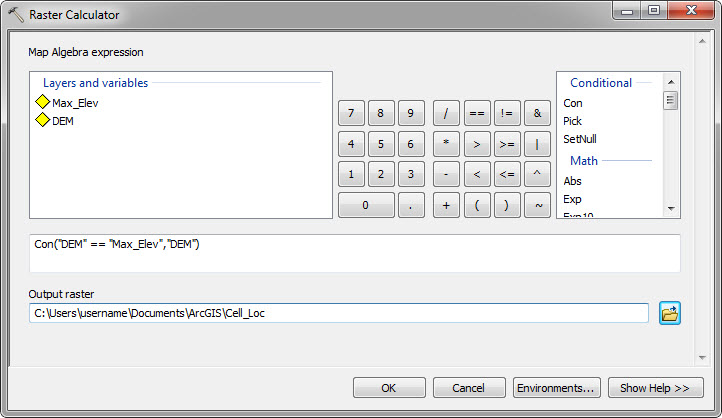English

# How To: Create points representing the highest or lowest elevations within polygon features

## Summary

Using a Digital Elevation Model (DEM) raster and the Spatial Analyst extension, the location of the highest or lowest elevation can be identified for polygon features of interest. This procedure describes a basic workflow that generates a point feature class of the highest point within each polygon feature. In this example, highest elevations are used, but method this also works for lowest elevations.

## Procedure

The three general steps of the procedure are to identify the maximum elevation values within each polygon, identify which specific cells in the DEM correspond to the maximum values, and then convert those cells to a point feature class.

```Note:
A polygon feature class, a DEM, and the Spatial Analyst extension are required for this procedure.```
1. Identify the value of the highest elevation within each polygon feature using the Zonal Statistics tool: Open ArcMap and navigate to ArcToolbox > Spatial Analyst Tools > Zonal > Zonal Statistics. This generates a new raster that changes all the cell values in each polygon feature to the value of the highest elevation within that polygon feature. For example purposes, name this raster 'Max_Elev.' The input and output parameters are as follows:
1. The Input raster or feature zone data is the polygon feature class.
2. The Zone field is a field in the polygon feature class that contains a unique value for each polygon feature, such as OBJECTID.
3. The Input value raster is the DEM.
4. Select a name (Max_Elev) and location for the Output raster generated.
5. For Statistics type, select Maximum.
6. Make sure that Ignore NoData in calculations is checked.
2. Compare the DEM to the Max_Elev raster to determine which cells in the DEM correspond to the highest elevation found within each polygon feature. This is done using a conditional statement in the Raster Calculator: ArcToolbox > Spatial Analyst Tools > Map Algebra Raster Calculator. The output is a raster depicting only the DEM cells with the highest elevation values and all other cells set to NoData. The input and output of the Raster Calculator is as follows:
1. Use the following conditional statement, which states that if a cell in a DEM is equal to the corresponding cell in Max_Elev, then set the cell value of the output raster to what is in DEM. If the cell value in the DEM is not equal to the value in Max_Elev, it is set to NoData in the output raster.
```Con("DEM" == "Max_Elev","DEM")
```1. Provide a location and name for the Output raster. For example, 'Cell_Loc'.
```Note:
The output raster, Cell_Loc, may appear to not display but this is because only the cells with the highest elevations actually contain values.  These cells may be barely visible until zoomed in.  The remaining cells contain NoData values and therefore by default are not symbolized.```
1. Convert the Cell_Loc raster to a point feature class using the Raster to Point tool: ArcToolbox > Conversion Tools > From Raster > Raster to Point. The input and output parameters are as follows:
1. The Input raster is Cell_Loc.
2. The Field is 'Value'. This carries over the elevation value from the cell to the point feature.
3. Select a name and location for the Output point features.
```Note:
After the tool completes it may be necessary to change the symbology of the output point features so they are clearly visible.```